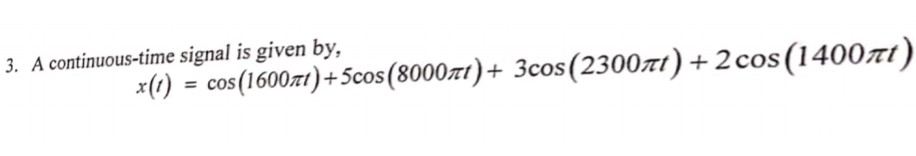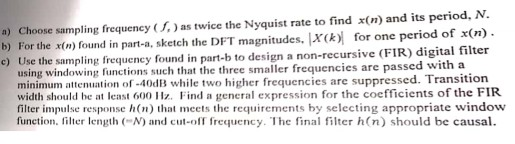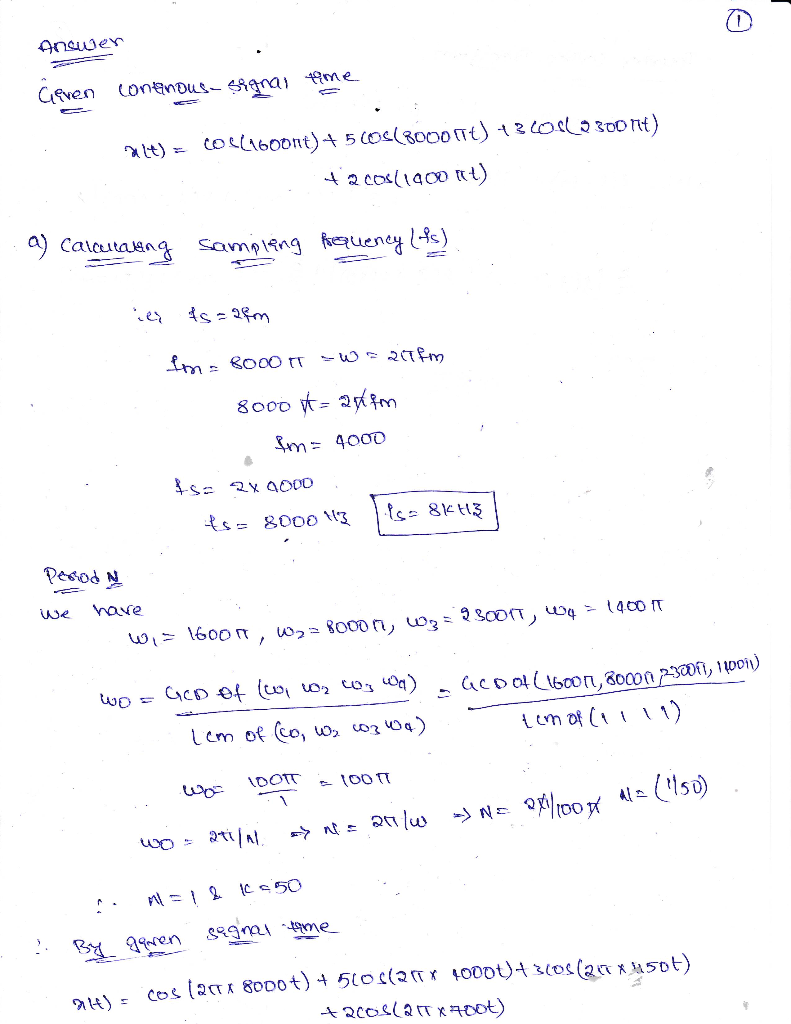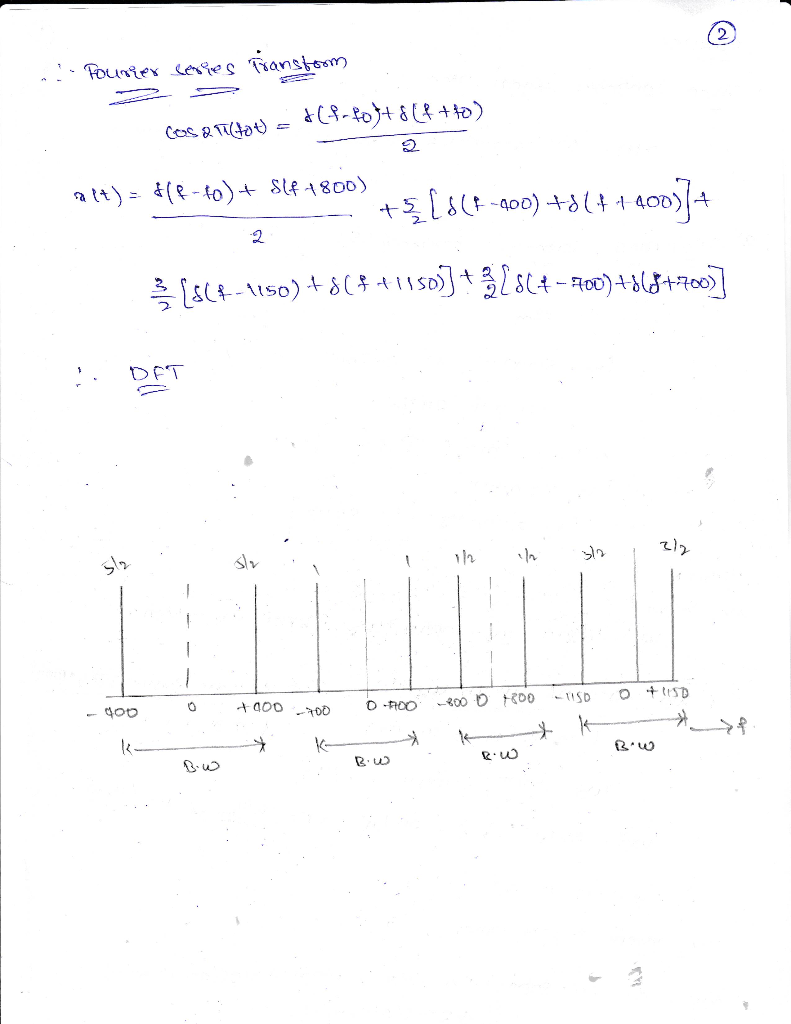# . A continuous-time signal is given by, x(r) = cos(1600m) +5cos(8000m)+ 3cos(2300m) +2cos(1400π .... A continuous-time signal is given by, x(r) = cos(1600m) +5cos(8000m)+ 3cos(2300m) +2cos(1400π
a) Choose sampling frequeney (S,) as twice the Nyquist rate to find x(n) and its period, N. magnitudes, X(k)for one period of x(n. in part-b to design a non-recursive (FIR) digital filter b) For the xen) ound in part-a, sketch the DFT o) usin the hamwline frergueney found in parth to desiegn a non-recursive (FIR) digital fiter using windowing functions such that the three smaller frequencies are minimum attenuation of-40dB while two higher frequencies are suppressed. Transition width should be at least 600z. Find a general expression for the coefficients of the FIR filter impulse response h(n) that meets the requirements by selecting appropriate window function, filter length N) and ctlf frequency. The final filter h(n) should be causal.##### Add Answer of: . A continuous-time signal is given by, x(r) = cos(1600m) +5cos(8000m)+ 3cos(2300m) +2cos(1400π ...
More Homework Help Questions Additional questions in this topic.